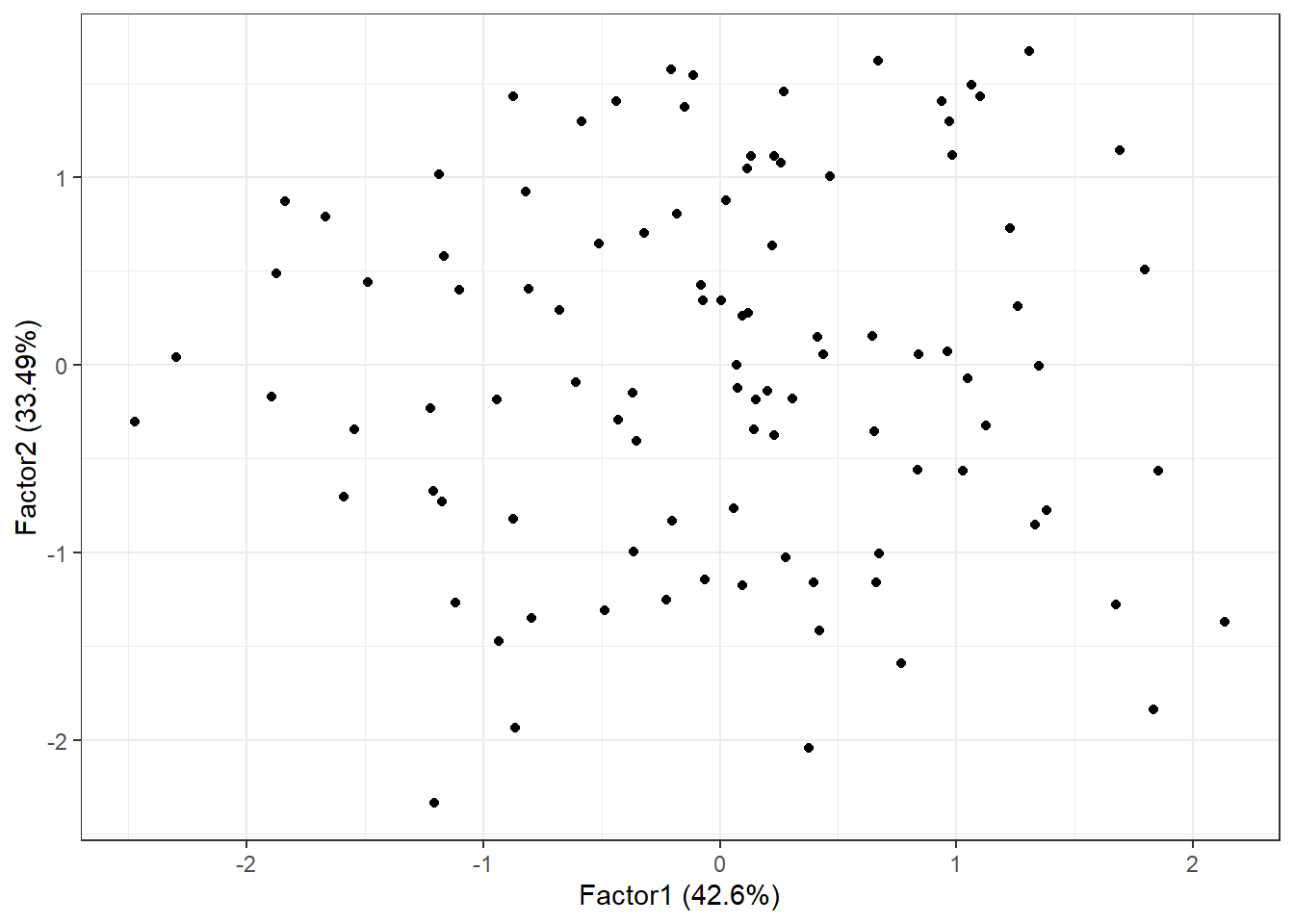## 15.6 Factor Scores

We could obtain Factor scores for an individual based on only the X’s that highly load on that factor Essentially here, FA would identify subgroups of correlated variables.

In our hypothetical example where after rotation x1 and x2 loaded highly on factor 2, and x3-5 loaded highly on factor 1, we could calculate factor scores as

• factor score 2 for person $$i$$ = $$x_{i1} + x_{i2}$$
• factor score 3 for person $$i$$ = $$x_{i3} + x_{i4} + x_{i5}$$

In some simple applications, this approach may be sufficient.

More commonly, we will use a regression procedure to compute factor scores. This method accounts for the correlation between the $$x_{i}$$’s and uses the factor loadings $$l_{ij}$$ to calculate the factor scores.

• Can be used as dependent or independent variables in other analyses
• Can be generated by adding the scores="regression" option to factanal(), or scores=TRUE in principal()
• Each record in the data set with no missing data will have a corresponding factor score.
• principal() also has a missing argument that if set to TRUE it will impute missing values.
fa.ml.varimax <- factanal(stan.dta, factors=2, rotation="varimax", scores="regression")
summary(fa.ml.varimax$scores) ## Factor1 Factor2 ## Min. :-2.47094 Min. :-2.335593 ## 1st Qu.:-0.70659 1st Qu.:-0.737829 ## Median : 0.08397 Median :-0.002978 ## Mean : 0.00000 Mean : 0.000000 ## 3rd Qu.: 0.67114 3rd Qu.: 0.792273 ## Max. : 2.13449 Max. : 1.670956 head(fa.ml.varimax$scores)
##         Factor1     Factor2
## [1,]  0.9713019  1.29838695
## [2,] -1.1676730  0.57888326
## [3,] -0.6068270 -0.09329792
## [4,]  1.2569753  0.31231783
## [5,]  1.3817494 -0.77707241
## [6,]  0.2311359  1.11142513
#library(ggforitfy)
autoplot(fa.ml.varimax) # see vignette for more info. Link at bottomTo merge these scores back onto the original data set providing there is no missing data you can use the bind_cols() function in dplyr.

data.withscores <- bind_cols(data, data.frame(fa.ml.varimax\$scores))
kable(head(data.withscores))
X1 X2 X3 X4 X5 Factor1 Factor2
0.5989843 1.3729499 1.0871992 0.8597854 1.2485892 0.9713019 1.2983869
0.7429206 0.3819301 -0.0113714 -1.1316383 -1.1316216 -1.1676730 0.5788833
-0.5699028 -0.1331253 -0.3594030 -0.7153705 -0.7274903 -0.6068270 -0.0932979
1.6526585 0.2216533 0.6564431 1.4564378 1.1959989 1.2569753 0.3123178
-0.8582815 -0.6310620 1.2474978 1.2291103 1.0684566 1.3817494 -0.7770724
1.1682966 0.9849325 -0.8860830 -0.0713417 0.5105760 0.2311359 1.1114251